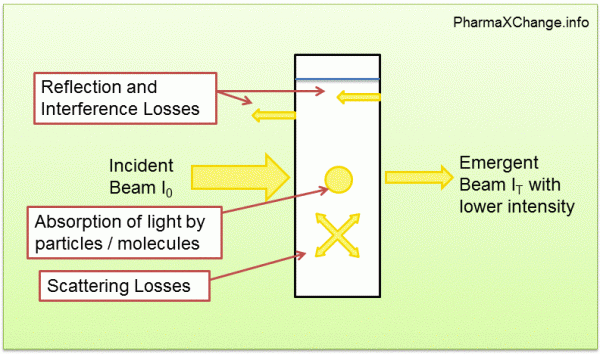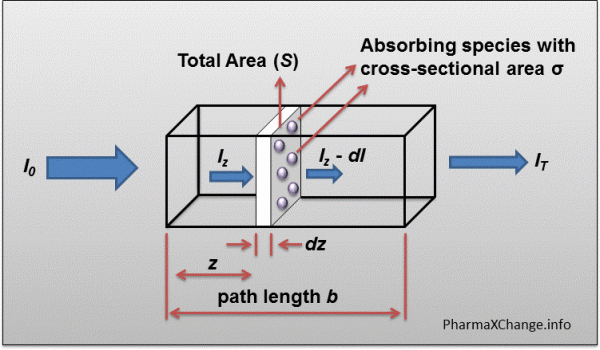# Ultraviolet-Visible (UV-Vis) Spectroscopy – Derivation of Beer-Lambert Law

Beer-Lambert law helps to correlate the intensity of absorption of UV-visible radiation to the amount of substance presence in a sample. Beer-Lambert law has been widely used in many fields of pharmaceutical sciences, chemistry and quantification testing. It allows UV-visible spectroscopy to be useful as not just a qualitative but also a quantitative tool.

## Derivation of Beer-Lambert Law

As has been seen in Ultraviolet-Visible (UV-Vis) Spectroscopy – Principle, light absorption of ultraviolet and visible light occurs due to absorption of energy by electrons which can be excited (such as π-electrons). Practically when a beam of light hits a substance, the machine can measure the intensity or power of light before it strikes the substance (I0), and then after it goes through the substance (IT). The incident light may undergo absorption, reflection, interference, and scattering before it is transmitted. Thus the intensity of light through the substance (IT) will be reduced.Figure showing what happens to light as it passes through a solution held in a container. The incident light is reduced in intensity due to absorption, reflection, interference and scattering of light.

The change in intensity of light due to absorption, reflection, interference and scattering, can therefore be equated as:

ΔI = I0IT

where I0 is the intensity of incident light, IT is the intensity of light transmitted and ΔI is the change in intensity of light due to absorption, reflection, interference and scattering by the solution.
i.e. ΔI = Iabsorbed + Ireflected + Iinterference + Iscattering

Normally, in order to measure just the amount of light that is absorbed by the sample, we keep an appropriate control sample with just the solvent without any dissolved solute. In such a case
ΔI = Iabsorbed

The ratio of the light passing through a substance is measured as transmittance which can be calculated as IT/I0. The % transmittance is therefore calculated as:

Absorbance is defined as the amount of light which is absorbed by the substance and is calculated as the negative logarithm of transmittance:

## History of the Beer-Lambert Law and Classical Derivations

### Lambert Derivation

In 1760, Lambert stated that the rate of decrease in the intensity of light with thickness (b) of the medium is proportional to the intensity of the incident light. When expressed mathematically:

where k’ is the proportionality constant, therefore when we take the reciprocal of the entire equation:

Integrating the above equation we get:

where IT is the intensity transmitted at thickness b and C is the constant of integration.

Now in order to solve for the constant of integration C, consider the case where b=0 (i.e. the thickness of the substance is 0). In such a case IT would be = I0. In such a case the equation would breakdown to give us:

Therefore if this value of C may be substituted in the above equation as:

Since it is normal to convert to common log10 we get:

By definition, the left side of this equation (i.e. log I0 / IT ) is nothing but absorbance (A). Therefore the equaation then becomes:

Thus Lambert’s Law was defined as: The intensity of parallel monochromatic radiation decreases exponentially as it passes through a medium of homogenous thickness. Simply put, the absorbance is proportional to the thickness ( path length b) of the solution.

### Beer Derivation

In 1852, in a similar derivation to Lambert’s, Beer demonstrated the following relationship between absorbance and concentration of a solution:

where, kn is a proportionality constant, c is the concentration and A is the absorbance.

Thus, Beer’s law can be defined as: the intensity of a beam of parallel monochromatic radiation decreases exponentially with the number of absorbing molecules. More simply, it stated that absorbance is proportional to the concentration (c) of the solution.

A combination of the above two laws i.e. the Lambert law and Beer’s law yields the modern derivation of the Beer-Lambert law.

## Modern Derivation of the Beer-Lambert Law

The modern derivation of the Beer-Lambert law combines the two derivations in order to relate absorbance of a substance to both the concentration and the path length of the sample.

Note: A lot of websites have not explained this derivation well in terms of what is actually happening. I will attempt to simplify it by explaining each step carefully and give definitions which are important to know rather than jumping from one equation to another. I would recommend reading this at least two times in order to understand what is going on. Please feel free to comment below in case you have any doubts on this.A diagrammatic representation of the hypothetical situation to derive the Beer-Lambert law containing a sample of path length b through which monochromatic light is passed.

Consider a beam of parallel monochromatic light with intensity I0 striking the sample at the surface as shown in the figure above, such that it is perpendicular to the surface. After passing through the path length b of the sample, which contains N molecules/cm3, the intensity of the light reduces to IT.

Now, consider a cross-section of the block having an area S and an infinitesimal thickness dz placed at a distance z from the surface.

If the sample has N molecules/cm3, the number of molecules present in the infinitesimal block would be
= N × S × dz

If each molecule has a cross-sectional area σ, where photons of light get absorbed, then the fraction of the total area where light gets absorbed due to each molecule would be (fractional area for each molecule)
= σ / S.

The total fractional area for all molecules in the block where light gets absorbed would therefore be
= (number of molecules) × (fractional area for each molecule)
i.e.= (N × S × dz) x (σ / S)  = σ × N × dz

If Iz is the light entering the infinitesimal block considered, and the light absorbed due to the absorbing particles is dI, the light exiting the slab would be IzdI.
The fraction of light absorbed would therefore be = dI/Iz

Now, since the fractional area is the probability of light striking a molecule,
the fraction of light absorbed in the block = fractional area occupied by all the molecules in the slab.
i.e. dI / Iz =  – σ × N × dz
Note: The negative sign is placed to indicate absorption of light.

If we integrate this infinitesimal block for the whole sample from z=0 to z=b, where b is the path length of the entire solution we get

ln IT – ln I0 = – σ × N × b
OR
– ln ( IT I0) = σ × N × b

One can correlate the number of molecules/cm3 of sample to the concentration of the sample in moles/liter using the relationship:
c = N × 1000/ (6.023 × 1023)
OR
N = (6.023 × 10 23) × c / 1000 = 6.023 × 1020 x c

where c is the concentration in moles/litre, N is the number of molecules/cm3, and (6.023 x 1023) is the Avagadro’s number. N × 1000 would be therefore molecules / liter as 1 liter = 1000 cm3.

Substituting this term for N in the above equation we get:
– ln (IT / I0 ) =  σ × 6.023 × 1020 × c × b

To simplify this equation further we convert to log using the term 2.303 × log (x) = ln (x).
therefore we get,
– log (IT / I0) =  (σ × 6.023 × 1020 × c × b) / 2.303
OR
log (I0 / IT) = [ ( σ × 6.023 × 1020)/2.303 ] × c × b

The term log (I0IT) is nothing but absorbance (A). While the term in the brackets [ ] is a constant and can be replaced by a constant ε = ( σ × 6.023 × 1020)/2.303. Thus we get:
A =  ε × c × b
OR
A = εbc

Since we have used moles/liter as the units of concentration, ε stands for molar absorptivity (or molar extinction coefficient). If the units of concentration were g/liter then the ε would be replaced by “a” (absorptivity).

By definition, the molar absorptivity (ε) at a specified wavelength of a substance in solution is the absorbance at that wavelength of a 1 mole/liter solution of the substance in a cell having a path length of 1 cm. The units of molar absorptivity are liter mole-1 cm-1

Specific absorbance is the absorbance of a substance in solution of specific concentration in a cell of specific path length. The most commonly used term for specific absorbance is A1%1cm, which is the absorbance of a 1 g/100ml (1%) solution in a 1cm cell at a particular wavelength of light. The beer-lambert equation in such case becomes:
A = A1%1cmbc

where, c is the concentration measured in g/dl and b is the path length measured in 1cm.
Note: Generally the path length of most experiments performed is 1cm.

## Books on Analytical Chemistry and Spectroscopy

Check out these good books for analytical chemistry and spectroscopy

## References

1. Analytical Chemistry: An Introduction (Saunders Golden Sunburst Series) 7th Ed., by Douglas A. Skoog, Donald M. West, F. James Holler. 1999.
2. Practical Pharmaceutical Chemistry 4th Ed. Edited by A. H. Beckett and J. B. Stenlake. 1988.
3. Fundamentals of Analytical Chemistry 8th Ed., by Douglas A. Skoog, Donald M. West, F. James Holler, Stanley R. Crouch. 2003.

#### Related Posts

1.2.•3.•4.•